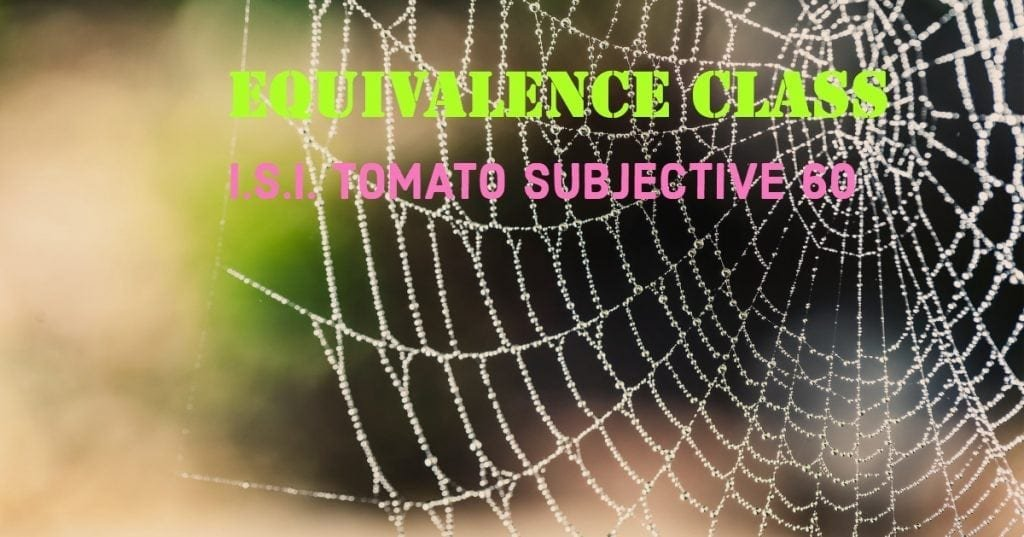How Cheenta works to ensure student success?
Explore the Back-Story

# Test of Mathematics Solution Subjective 60 - Equivalence ClassThis is a Test of Mathematics Solution Subjective 60 (from ISI Entrance). The book, Test of Mathematics at 10+2 Level is Published by East West Press. This problem book is indispensable for the preparation of I.S.I. B.Stat and B.Math Entrance.

Also visit: I.S.I. & C.M.I. Entrance Course of Cheenta

## Problem

Consider the set S of all integers between and including 1000 and 99999. Call two integers x and y in S to be in the same equivalence class if the digits appearing in x and y are the same. For example, if x = 1010, y = 1000 and z = 1201, then x and y are in the same equivalence class, but y and z are not. Find the number of distinct equivalence classes that can be formed out of S.

## Solution

Any set of distinct digits with maximum order 5 is a equivalence class that can be formed out of S except {0}.
Number of such sets is
= ${ {10} \choose {1}}$ + ${ {10} \choose {2}}$ + ${{10} \choose {3}}$ + ${ {10}\choose{4}}$ + ${{10}\choose{5}}$ -1
= 10 + 45 + 120 + 210 + 252 -1
= 636This is a Test of Mathematics Solution Subjective 60 (from ISI Entrance). The book, Test of Mathematics at 10+2 Level is Published by East West Press. This problem book is indispensable for the preparation of I.S.I. B.Stat and B.Math Entrance.

Also visit: I.S.I. & C.M.I. Entrance Course of Cheenta

## Problem

Consider the set S of all integers between and including 1000 and 99999. Call two integers x and y in S to be in the same equivalence class if the digits appearing in x and y are the same. For example, if x = 1010, y = 1000 and z = 1201, then x and y are in the same equivalence class, but y and z are not. Find the number of distinct equivalence classes that can be formed out of S.

## Solution

Any set of distinct digits with maximum order 5 is a equivalence class that can be formed out of S except {0}.
Number of such sets is
= ${ {10} \choose {1}}$ + ${ {10} \choose {2}}$ + ${{10} \choose {3}}$ + ${ {10}\choose{4}}$ + ${{10}\choose{5}}$ -1
= 10 + 45 + 120 + 210 + 252 -1
= 636

### Knowledge Partner